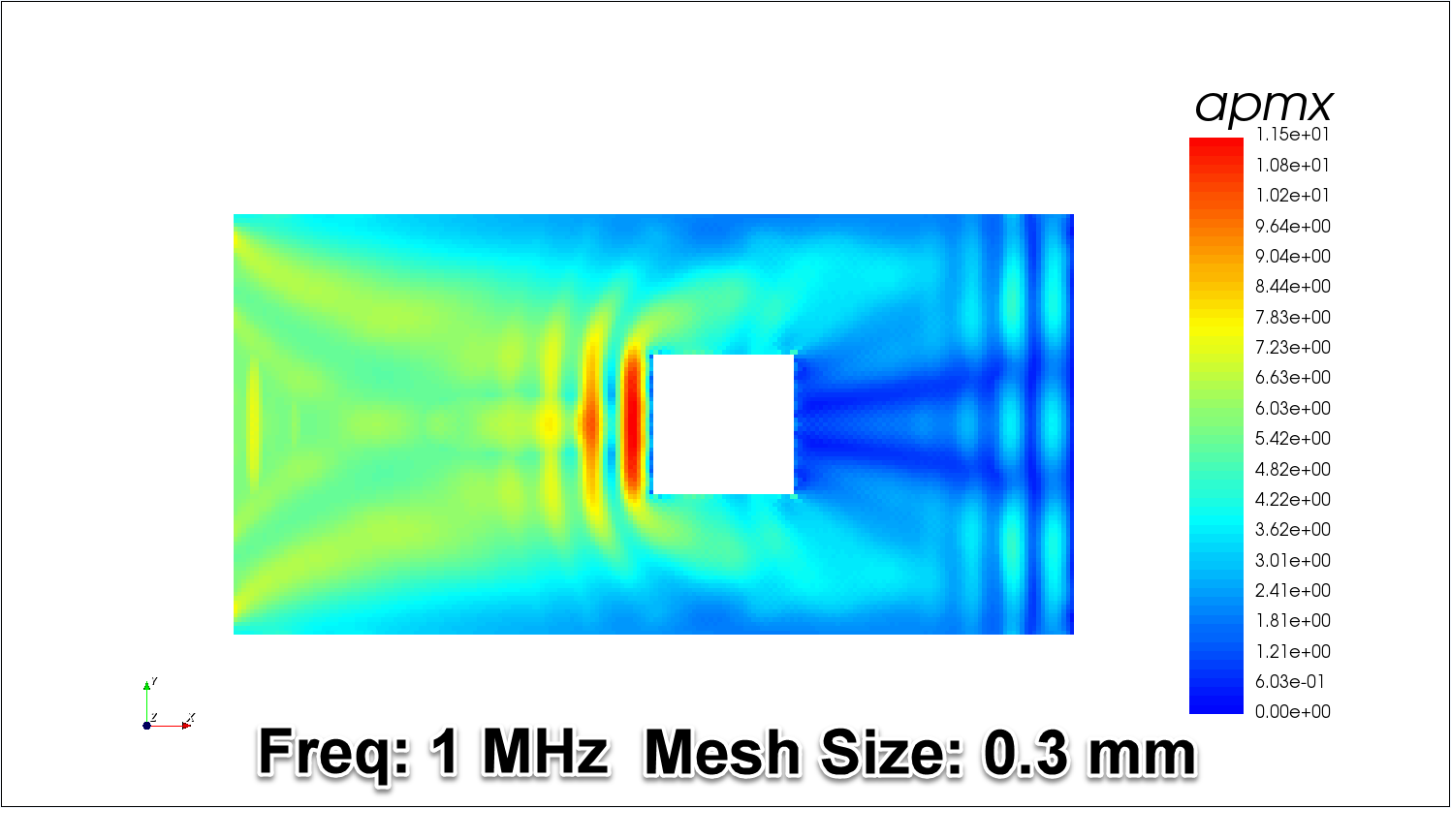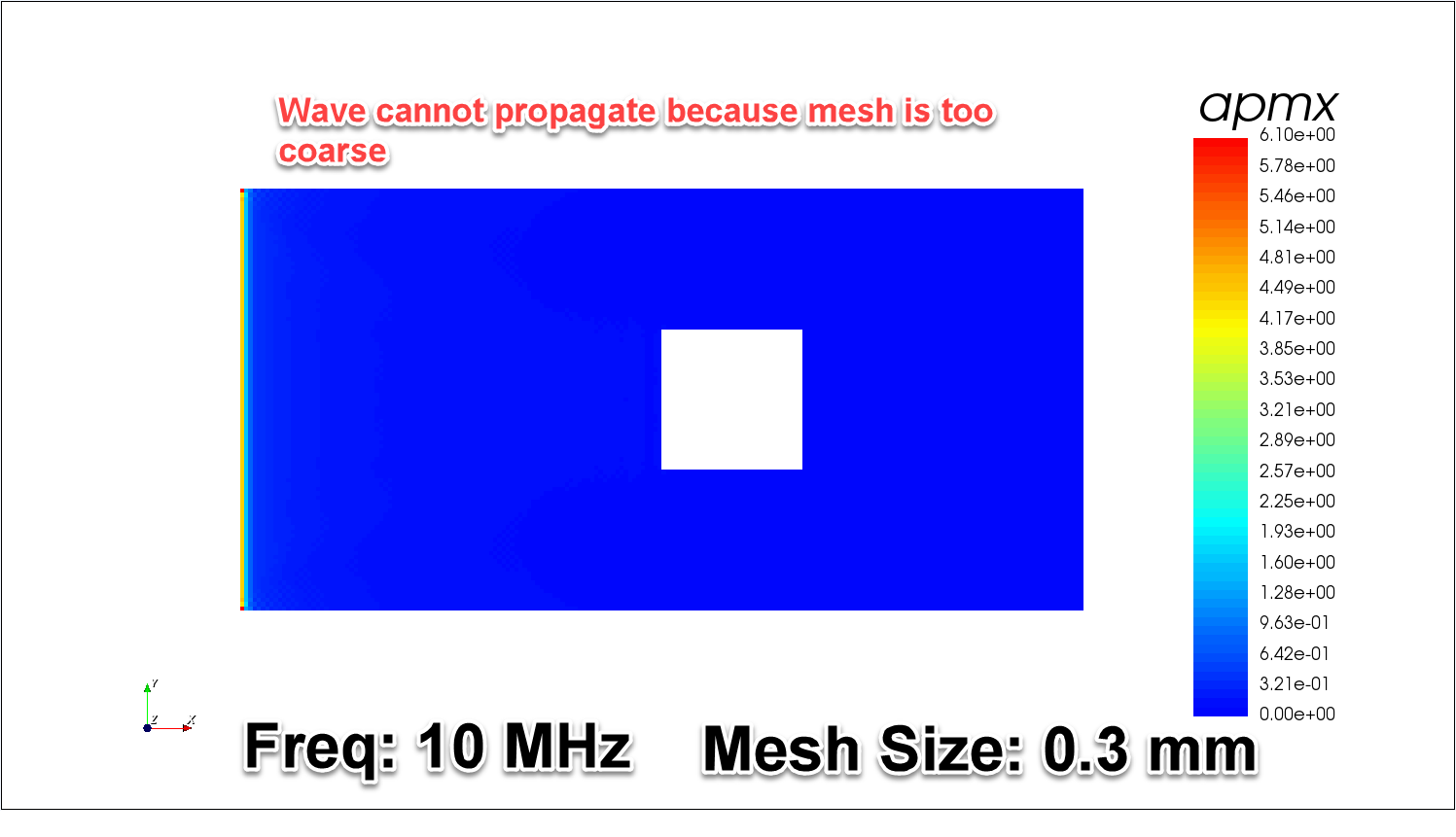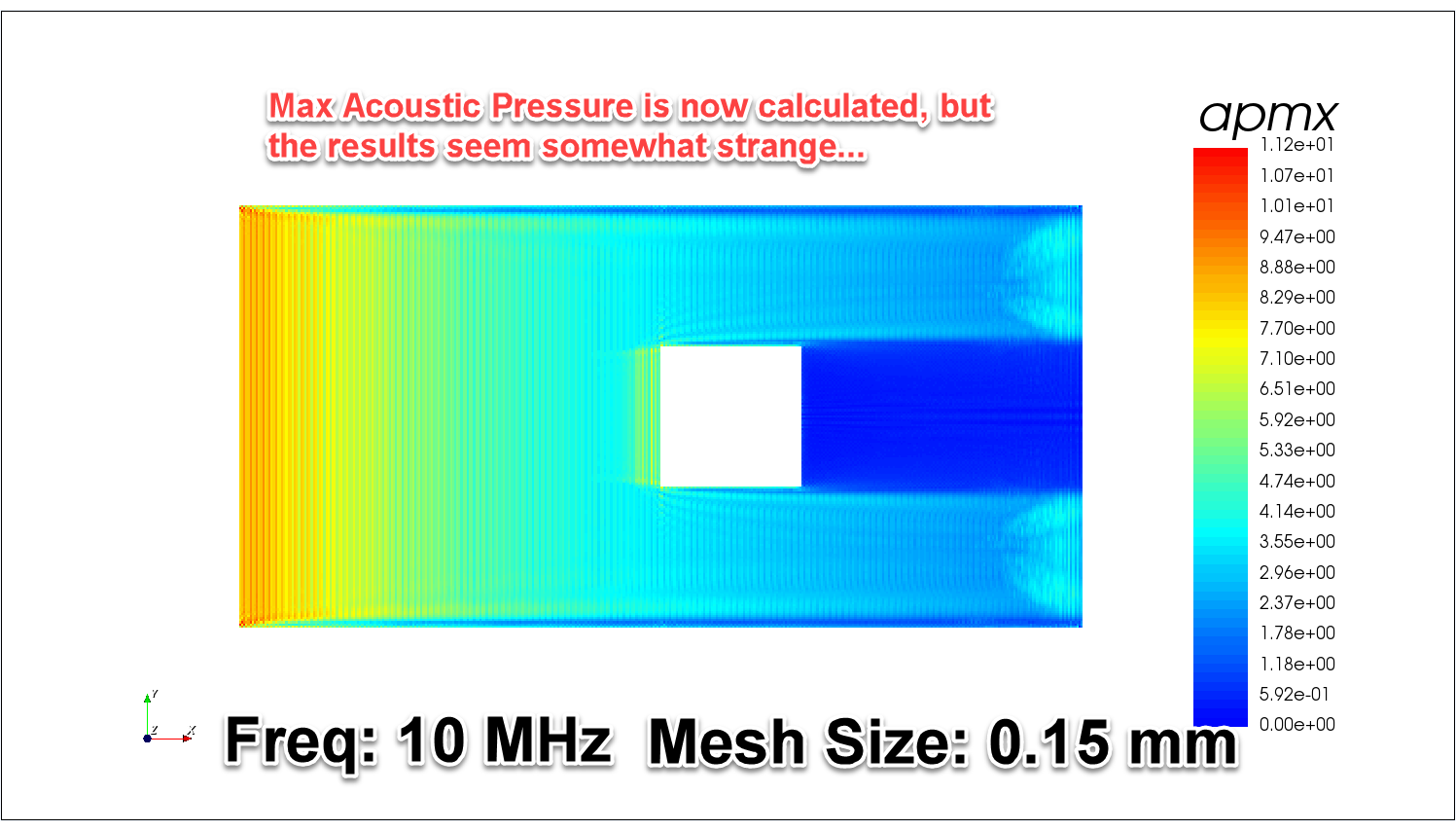# If the frequency of my transducer changes from 1 MHz to 10 MHz, should I also change the mesh size?

Question:

My transducer can transmit ultrasound which has 10 MHz to 1 MHz frequency.

I have to compare pressure and shear stress from 1 MHz to 10 MHz. Do I have to use a mesh size of 15 elements per wavelength? Or can I use a fixed mesh size at all frequencies?

If you change the frequency, you need to change the mesh too.

Frequency is inversely proportional to wavelength therefore increasing frequency will decrease the wavelength.

We recommend an element size of at least 1/15 of the smallest wavelength in the model.

Therefore a wave with a smaller wavelength (at a higher frequency) will require smaller elements to be accurately calculated.

This is the code typically used in OnScale to calculate the mesh size (\$box):

```symb freqint = 1e+06                   /* Determined Drive Frequency (Hz)
symb freqdamp = \$freqint
symb wavevel = 1179.0                  /* Longitudinal wave velocity in material (m/s)
symb wavelgth = \$wavevel / \$freqint    /* Wavelength of sound in material (m)
symb nmesh = 30                        /* Elements per wavelength
symb box = \$wavelgth / \$nmesh          /* Element size
```

As you can see, when the \$freqint increases, the \$box decreases.

Note: See related article: How meshing relates to wavelength in OnScale.

You can think about it like this:

A wave which has higher frequency travels faster … thus the wavelength of this wave is smaller.

To get good accuracy, divide the wavelength by 15 and you get the mesh size.

Compare the following results of max acoustic pressure (apmx) of propagation of a wave in a 2D steel plate with a hole:Note that this change in mesh size is required by the nonlinear explicit time solver that OnScale uses. If mesh size is too coarse, the CFL condition will not be verified!

The advantage of higher frequency is that the total simulation time is shorter because the wave travels faster. Lower frequency can have coarser mesh, but you may need to set up a longer total simulation time in order to capture the whole discharge of the electrode. (This is required to calculate the impedance in the frequency domain).